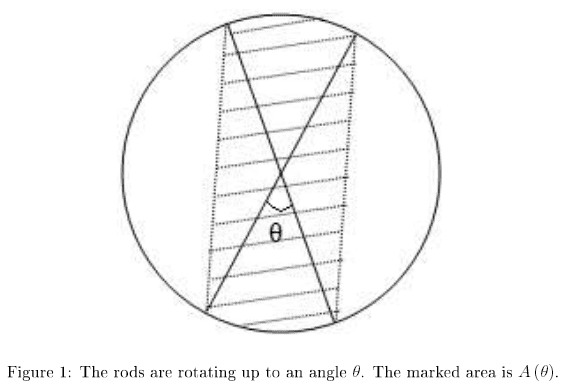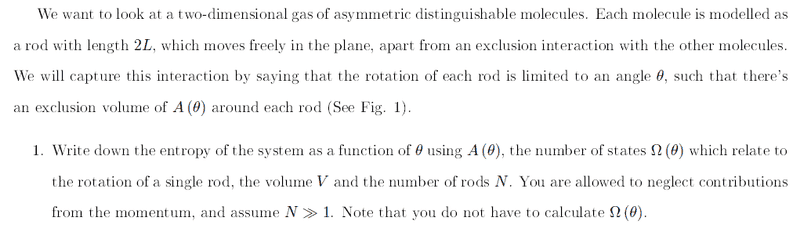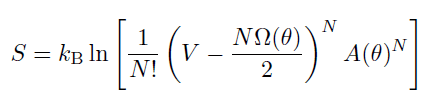# Entropy of translation and rotation of a molecules

## Homework Statement

Hi all,
There is a question from the course book:## Homework Equations

$$S=k_B ln W$$

## The Attempt at a Solution

My solution:
So first of all, for each molecule, there are 2 motions: translational and rotational.
For rotational I get:
$$W_1 =\Omega \left ( \theta \right )$$
For translational, I should calculate the free (non forbidden volume) for molecule to move in. Since the overall volume is V and there are N molecules, the volume allowed for moving is:
$$W_2 =V-NA \left ( \theta \right )$$
Since molecules are distinguishable, I need to divide by (N!)^(1/N) for each molecule, so:
$$W_2 =\frac{V-NA \left ( \theta \right )}{\sqrt[N]{N!}}$$
Therefore, the entropy per molecule is:
$$s= k_B ln \left ( \frac{V-NA \left ( \theta \right )}{\sqrt[N]{N!}}\Omega \left ( \theta \right ) \right )$$
And since there are N molecules:
$$S= k_B N ln \left ( \frac{V-NA \left ( \theta \right )}{\sqrt[N]{N!}}\Omega \left ( \theta \right ) \right )=k_B ln \left (\frac{1}{N!} \left ( V-NA \left ( \theta \right ) \right )^N \left ( \Omega \left ( \theta \right ) \right )^N \right )$$

But according the book, the answer is:So I have 2 questions:
1. Do they mixed up between Omega and A? Or it's me?
2. Where do a factor of 2 came from in the expression for the volume?

Thanks!